## Maharashtra State Board Class 7 Maths Solutions | Maths Digest Std 7

Expert Teachers has created Maharashtra State Board Class 7 Maths Solutions Digest Pdf Download. You can also Download 7th Class Maths Book Solutions Maharashtra Board to help you to revise the complete Syllabus and score more marks in your examinations.

## Maths Solution Class 7 Maharashtra Board | 7th Standard Maths Digest

7th Std Maths Solution Maharashtra Board Chapter 1 Geometrical Constructions

Practice Set 7th Class Maharashtra State Board Chapter 2 Multiplication and Division of Integers

Class 7 Maths Solution Maharashtra Board Chapter 3 HCF and LCM

Class 7 Maths State Board Chapter 4 Angles and Pairs of Angles

Std 7 Maths Textbook Maharashtra Board Chapter 5 Operations on Rational Numbers

Class 7 Maths Maharashtra Board Chapter 6 Indices

Std 7 Maths Maharashtra Board Chapter 7 Joint Bar Graph

7th Maths Practice Set 32, 33, 34, 35 and 36 Chapter 8 Algebraic Expressions and Operations on them

Maharashtra Board Class 7 Maths Miscellaneous Problems Set 1

7th Standard Practice Set 37, 38 and 39 Direct Proportion and Inverse Proportion Chapter 9

Bank and Simple Interest Std 7 Practice Set 40 and 41 Chapter 10

7th Standard Maths Practice Set 42 and 43 Chapter 11 Circle

Class 7 Math Book Solution Chapter 12 Perimeter and Area

Maharashtra State Board Class 7 Maths Chapter 13 Pythagoras Theorem

Std 7 Maths Practice Set Chapter 14 Algebraic Formulae – Expansion of Squares

7th Standard Maths Maharashtra Board Chapter 15 Statistics

Maharashtra Board Class 7 Maths Miscellaneous Problems Set 2

Maharashtra State Board Class 7 Textbook Solutions

## Maharashtra State Board Class 8 Maths Solutions | Maths Digest Std 8

Expert Teachers has created Maharashtra State Board Class 8 Maths Solutions Digest Pdf Download. You can also Download Maths Solution Class 8 Maharashtra Board to help you to revise the complete Syllabus and score more marks in your examinations.

## Maths Solution Class 8 Maharashtra Board | 8th Standard Maths Digest

Maharashtra Board Class 8 Maths Chapter 1 Rational and Irrational Numbers

Maharashtra Board Class 8 Maths Chapter 2 Parallel Lines and Transversals

Maharashtra Board Class 8 Maths Chapter 3 Indices and Cube Root

Maharashtra Board Class 8 Maths Chapter 4 Altitudes and Medians of a Triangle

Maharashtra Board Class 8 Maths Chapter 5 Expansion Formulae

Maharashtra Board Class 8 Maths Chapter 6 Factorisation of Algebraic Expressions

Maharashtra Board Class 8 Maths Chapter 7 Variation

Maharashtra Board Class 8 Maths Chapter 8 Quadrilateral: Constructions and Types

Maharashtra Board Class 8 Maths Chapter 9 Discount and Commission

Maharashtra Board Class 8 Maths Miscellaneous Exercise 1

Maharashtra Board Class 8 Maths Chapter 10 Division of Polynomials

Maharashtra Board Class 8 Maths Chapter 11 Statistics

Maharashtra Board Class 8 Maths Chapter 12 Equations in One Variable

Maharashtra Board Class 8 Maths Chapter 13 Congruence of Triangles

Maharashtra Board Class 8 Maths Chapter 14 Compound Interest

Maharashtra Board Class 8 Maths Chapter 15 Area

Maharashtra Board Class 8 Maths Chapter 16 Surface Area and Volume

Maharashtra Board Class 8 Maths Chapter 17 Circle: Chord and Arc

Maharashtra Board Class 8 Maths Miscellaneous Exercise 2

Maharashtra State Board Class 8 Textbook Solutions

## Maharashtra State Board Class 9 Maths Solutions Digest Part 1 & 2

Expert Teachers has created Maharashtra State Board Class 9 Maths Solutions Algebra Geometry Part 1 & 2 Pdf. You can also Download 9th Class Maths Solution Maharashtra Board to help you to revise complete Syllabus and score more marks in your examinations.

Students can also check out the Maharashtra State Board Class 9 English Solutions & Maharashtra State Board Class 9 History Solutions & Maharashtra State Board Class 9 Science Solutions here that will help you prepare for the exams.

## 9th Class Maths Solution Maharashtra Board

### Maharashtra State Board 9th Maths Solutions Part 1 Algebra Pdf

Maths 1 Digest Std 9

Maharashtra Board Class 9 Maths Chapter 1 Sets

Maths Solution Class 9 Maharashtra Board Chapter 2 Real Numbers

Maharashtra State Board 9th Maths Solution Chapter 3 Polynomials

Class 9 Maths Solution Maharashtra Board Chapter 4 Ratio and Proportion

9th Class Maths Solution Maharashtra Board Chapter 5 Linear Equations in Two Variables

Maharashtra Board 9th Maths Solution Chapter 6 Financial Planning

Maharashtra Board Class 9 Maths Chapter 7 Statistics

### Maharashtra State Board 9th Maths Solutions Part 2 Geometry Pdf

Maths 2 Digest Std 9

Std 9 Maths Solutions Maharashtra State Board Chapter 1 Basic Concepts in Geometry

Maharashtra Board Class 9 Maths Chapter 2 Parallel Lines

Maharashtra Board Class 9 Maths Chapter 3 Triangles

Maharashtra Board Class 9 Maths Chapter 4 Constructions of Triangles

Maharashtra Board Class 9 Maths Chapter 5 Quadrilaterals

Maharashtra Board Class 9 Maths Chapter 6 Circle

Maharashtra Board Class 9 Maths Chapter 7 Co-ordinate Geometry

Maharashtra Board Class 9 Maths Chapter 8 Trigonometry

Maharashtra Board Class 9 Maths Chapter 9 Surface Area and Volume

Maharashtra State Board Class 9 Textbook Solutions

## Maharashtra State Board Class 6 Maths Solutions | Maths Digest Std 6

Expert Teachers has created Maharashtra State Board Class 6 Maths Solutions Digest Pdf Download. You can also Download Maths Solution Class 6 Maharashtra Board to help you to revise the complete Syllabus and score more marks in your examinations.

Students can also check out the Maharashtra State Board Class 6 Hindi Solutions & Maharashtra State Board Class 6 English Solutions & Maharashtra State Board Class 6 Science Solutions here that will help you prepare for the exams.

## Maths Solution Class 6 Maharashtra Board | 6th Standard Maths Digest

Maharashtra Board Class 6 Maths Chapter 1 Basic Concepts in Geometry

Maharashtra Board Class 6 Maths Chapter 2 Angles

Maharashtra Board Class 6 Maths Chapter 3 Integers

Maharashtra Board Class 6 Maths Chapter 4 Operations on Fractions

Maharashtra Board Class 6 Maths Chapter 5 Decimal Fractions

Maharashtra Board Class 6 Maths Chapter 6 Bar Graphs

Maharashtra Board Class 6 Maths Chapter 7 Symmetry

Maharashtra Board Class 6 Maths Chapter 8 Divisibility

Maharashtra Board Class 6 Maths Chapter 9 HCF-LCM

Maharashtra Board Class 6 Maths Chapter 10 Equations

Maharashtra Board Class 6 Maths Chapter 11 Ratio-Proportion

Maharashtra Board Class 6 Maths Chapter 12 Percentage

Maharashtra Board Class 6 Maths Chapter 13 Profit-Loss

Maharashtra Board Class 6 Maths Chapter 14 Banks and Simple Interest

Maharashtra Board Class 6 Maths Chapter 15 Triangles and their Properties

Maharashtra Board Class 6 Maths Chapter 16 Quadrilaterals

Maharashtra Board Class 6 Maths Chapter 17 Geometrical Constructions

Maharashtra Board Class 6 Maths Chapter 18 Three Dimensional Shapes

Maharashtra State Board Class 6 Textbook Solutions

## Maharashtra State Board Class 10 Maths Solutions Digest Part 1 & 2

Expert Teachers has created Maharashtra State Board Class 10 Maths Solutions Algebra Geometry Part 1 & 2 Pdf. You can also Download Solution of 10th Class Maths Maharashtra Board Pdf to help you to revise complete Syllabus and score more marks in your examinations.

### Maharashtra State Board Class 10 Maths Solutions Algebra Pdf Part 1

Problem Set 1 Geometry 10th Maharashtra Board Chapter 1 Linear Equations in Two Variables

Maharashtra State Board Class 10 Maths Solutions Chapter 2 Quadratic Equations

Problem Set 3 Algebra Class 10 Chapter 3 Arithmetic Progression

Financial Planning Class 10 Pdf Chapter 4

Practice Set 5.1 Geometry Class 10 Chapter 5 Probability

Problem Set 6 Algebra Class 10 Chapter 6 Statistics

### Maharashtra State Board Class 10 Maths Solutions Geometry Pdf Part 2

Maharashtra Board Class 10 Maths Chapter 1 Similarity

Maharashtra Board Class 10 Maths Chapter 2 Pythagoras Theorem

Maharashtra Board Class 10 Maths Chapter 3 Circle

Problem Set 6 Geometry Class 10 Chapter 4 Geometric Constructions

Maharashtra State Board Class 10 Maths Solutions Geometry Chapter 5 Co-ordinate Geometry

Trigonometry 10th Class Problem Set 6 Maharashtra Board Class 10 Maths Chapter 6

Problem Set 7 Geometry 10th Maharastra Board Chapter 7 Mensuration

Maharashtra State Board Class 10 Textbook Solutions

## Maharashtra State Board Class 10 Maths Solutions Chapter 4 Financial Planning Practice Set 4.2

Question 1. ‘Chetana Store’ paid total GST of ₹ 1,00,500 at the time of purchase and collected GST ₹ 1,22,500 at the time of sale during 1st of July 2017 to 31st July 2017. Find the GST payable by Chetana Stores.
Output tax (Tax collected at the time of sale)
= ₹ 1,22,500
Input tax (Tax paid at the time of purchase)
= ₹ 1,00,500
ITC (Input Tax credit) = ₹ 1,00,500.
GST payable = Output tax – ITC
= 1,22,500 – 1,00,500
= ₹ 22,000
GST payable by Chetana stores is ₹ 22,000.

Question 2. Nazama is a proprietor of a firm, registered under GST. She has paid GST of ₹ 12,500 on purchase and collected ₹ 14,750 on sale. What is the amount of ITC to be claimed? What is the amount of GST payable?
Solution:
Output tax = ₹ 14,750
Input tax = ₹ 12,500
∴ ITC for Nazama = ₹ 12,500.
∴ GST payable = Output tax – ITC
= 14750 – 12500
= ₹ 2250
∴ Amount of ITC to be claimed is ₹ 12,500 and amount of GST payable is ₹ 2250.

Question 3. Amir Enterprise purchased chocolate sauce bottles and paid GST of ₹ 3800. He sold those bottles to Akbari Bros, and collected GST of ₹ 4100. Mayank Food Corner purchased these bottles from Akbari Bros, and paid GST of ₹ 4500. Find the amount of GST payable at every stage of trading and hence find payable CGST and SGST.
Solution:
For Amir Enterprise:
Output tax = ₹ 4100
Input tax = ₹ 3800
ITC for Amir enterprise = ₹ 3800.
∴ GST payable = Output tax – ITC
= 4100 – 3800
= ₹ 300
For Akbari Bros.:
Output tax = ₹ 4500
Input tax = ₹ 4100
ITC for Akbari Bros = ₹ 4100.
GST payable = Output tax – ITC
= 4500 – 4100 = ₹ 400
∴ Statement of GST payable at every stage of trading: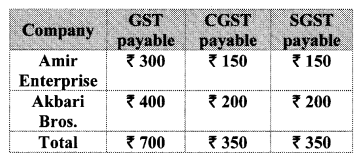Question 4. Malik Gas Agency (Chandigarh Union Territory) purchased some gas cylinders for industrial use for ₹ 24,500, and sold them to the local customers for ₹ 26,500. Find the GST to be paid at the rate of 5% and hence the CGST and UTGST to be paid for this transaction, (for Union Territories there is UTGST instead of SGST.)
Solution:
For Malik Gas Agency:
Output tax = 5% of 26500
= $$\frac { 5 }{ 100 }$$ × 26500
= ₹ 1325
Input tax = 5% of 24500
= $$\frac { 5 }{ 100 }$$ × 24500
= ₹ 1225
ITC for Malik Gas Agency = ₹ 1225.
∴ GST payable = Output tax – ITC
= 1325 – 1225
= ₹ 100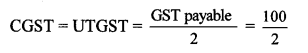∴ CGST = UTGST = ₹ 50
∴ The GST to be paid at the rate of 5% is ₹ 100 and hence, CGST and UTGST paid for the transaction is ₹ 50 each.

Question 5.
M/s Beauty Products paid 18% GST on cosmetics worth ₹ 6000 and sold to a customer for ₹ 10,000. What are the amounts of CGST and SGST shown in the tax invoice issued?
Solution:
Output tax = 18% of 10,000
= $$\frac { 18 }{ 100 }$$ × 10,000
= ₹ 1800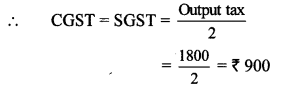∴ Amount of CGST and SGST shown in the tax invoice issued is ₹ 900 each.

Question 6.
Prepare Business to Consumer (B2C) tax invoice using given information. Write the name of the supplier, address, state, Date, Invoice number, GSTIN etc. as per your choice.
Supplier: M/s ______ Address _______ State _______ Date _______ Invoice No. _______ GSTIN _______
Particulars
Rate of Mobile Battery ₹ 200 Rate of GST 12% HSN 8507 1 PC
Rate of Headphone ₹750 Rate of GST 18% HSN 8518 1 Pc
Solution:
Rate of Mobile Battery = ₹200
CGST = 6% of 200
= $$\frac { 6 }{ 100 }$$ × 200
= ₹ 12
∴ CGST = SGST = ₹ 12

Rate of Headphone = ₹ 750
COST = 9% of 750
= $$\frac { 9 }{ 100 }$$ × 750
= ₹ 67.5
∴ CGST = SGST = ₹ 67.5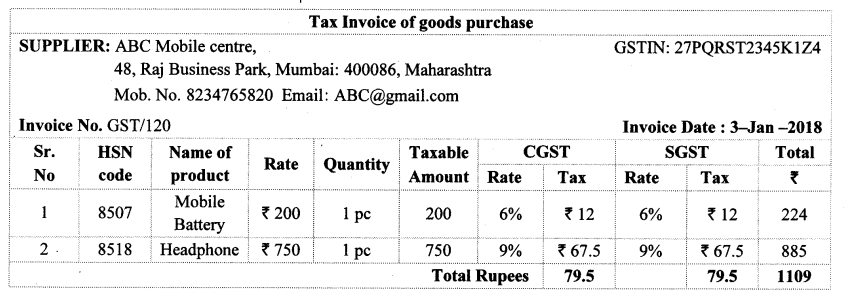Question 7.
Prepare Business to Business (B2B) Tax Invoice as per the details given below, name of the supplier, address, Date etc. as per your choice.
Supplier – Name, Address, State, GSTIN, Invoice No., Date
Recipient – Name, Address, State, GSTIN,
Items:
i. Pencil boxes 100, HSN – 3924, Rate – ₹ 20, GST 12%
ii. Jigsaw Puzzles 50, HSN 9503, Rate – ₹ 100 GST 12%.
Solution:
Cost of 100 Pencil boxes
= 20 × 1oo
= ₹ 2000
CGST = 6% of 2000
= $$\frac { 6 }{ 100 }$$ × 2000
= ₹ 120
∴ CGST = SGST = ₹ 120

Cost of 50 Jigsaw Puzzles = 100 × 50
= ₹ 5000
CGST = 6% of 5000
= $$\frac { 6 }{ 100 }$$ × 5000
= ₹ 300
CGST – SGST = ₹ 300Question 1.
Suppose a manufacturer sold a cycle for a taxable value of ₹ 4000 to the wholesaler. Wholesaler sold it to the retailer for ₹ 4800 (taxable value). Retailer sold it to a customer for ₹ 5200 (taxable value). Rate of GST is 12%. Complete the following activity to find the payable CGST and SGST at each stage of trading. (Textbook pg. no. 92)
Solution: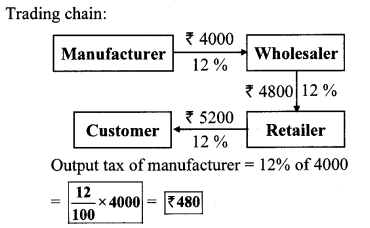GST payable by manufacturer = ₹ 480
Output tax of wholesaler
= 12% of 4800 = $$\frac { 12 }{ 100 }$$ × 4800 = ₹ 576
∴ GST payable by wholesaler
= Output tax – Input tax
= 576 – 480
= ₹ 96
Output tax of retailer = 12% of 5200Question 2. Suppose in the month of July the output tax of a trader is equal to the input tax, then what is his payable GST?(Textbook pg. no. 93)
Here, output tax is same as input tax.
∴ Trader payable GST will be zero.

Question 3.
Suppose in the month of July output tax of a trader is less than the input tax then how to compute his GST? (Textbook pg. no. 93)
If output tax of a trader in a particular month is less than his input tax, then he won’t be able to get entire credit for his input tax. In such a case his balance credit will be carried forward and adjusted against the subsequent transactions.

## Maharashtra State Board Class 10 Maths Solutions Chapter 4 Financial Planning Practice Set 4.3

Practice Set 4.3 Financial Planning Question 1. Complete the following table by writing suitable numbers and words.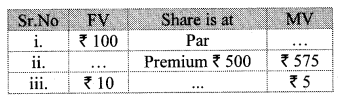Solution:
i. Here, share is at par.
∴ MV = FV
∴ MV = ₹ 100

ii. Here, Premium = ₹ 500, MV = ₹ 575
∴ FV + Premium = MV
∴ FV + 500 = 575
∴ FV = 575 – 500
∴ FV = ₹ 75

iii. Here, FV = ₹ 10, MV = ₹ 5
∴ FV > MV
Share is at discount.
FV – Discount = MV
∴ 10 – Discount = 5
∴ 10 – 5 = Discount
₹ Discount = ₹ 5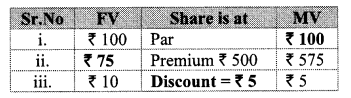Practice Set 4.3 Question 2. Mr. Amol purchased 50 shares of Face value ₹ 100 when the Market value of the share was ₹ 80. Company had given 20% dividend. Find the rate of return on investment.
Solution:
Here, MV = ₹ 80, FV = ₹ 100,
Number of shares = 50, Rate of dividend = 20%
∴ Sum invested = Number of shares × MV
= 50 × 80
= ₹ 4000

Dividend per share = 20% of FV
= $$\frac { 20 }{ 100 }$$ × 100 = ₹ 20
∴ Total dividend of 50 shares = 50 × 20
= ₹ 1000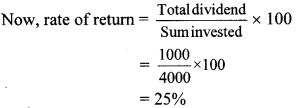∴ Rate of return on investment is 25%.

Question 3.
Joseph purchased following shares, Find his total investment.
Company A : 200 shares, FV = ₹ 2, Premium = ₹ 18.
Company B : 45 shares, MV = ₹ 500
Company C : 1 share, MV = ₹ 10,540
Solution:
For company A:
FV = ₹ 2, premium = ₹ 18,
Number of shares = 200
= 2 + 18
= ₹ 20
Sum invested = Number of shares × MV
= 200 × 20
= ₹ 14000

For company B:
MV = ₹ 500, Number of shares = 45
Sum invested = Number of shares × MV
= 45 × 500 = ₹ 22,500

For company C:
MV = ₹ 10,540, Number of shares = 1
∴ Sum invested = Number of shares × MV
= 1 × 10540
= ₹ 10,540
∴ Total investment of Joseph
= Investment for company A + Investment for company B + Investment for company C
= 4000 + 22,500 + 10,540
= ₹ 37040
∴ Total investment done by Joseph is ₹ 37,040.

Question 4.
Smt. Deshpande purchased shares of FV ₹ 5 at a premium of ₹ 20. How many shares will she get for ₹ 20,000?
Solution:
Here, FV = ₹ 5, Premium = ₹ 20,
Sum invested = ₹ 20,000
∴ MV = FV + Premium
= 5 + 20
∴ MV = ₹ 25
Now, sum invested = Number of shares × MV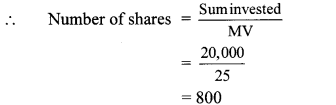∴ Smt. Deshpande got 800 shares for ₹ 20,000.

Question 5.
Shri Shantilal has purchased 150 shares of FV ₹ 100, for MV of ₹ 120. Company has paid dividend at 7%. Find the rate of return on his investment.
Solution:
Here, FV = ₹ 100, MV = ₹ 120
Dividend = 7%, Number of shares = 150
∴ Sum invested = Number of shares × MV
= 150 × 120 = ₹ 18000
Dividend per share = 7% of FV
= $$\frac { 7 }{ 100 }$$ × 100 = ₹ 7
∴ Total dividend of 150 shares
= 150 × 7 = ₹ 1050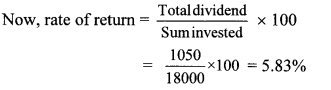∴ Rate of return on investment is 5.83%.

4.3 Class 10 Question 6. If the face value of both the shares is same, then which investment out of the following is more profitable?
Company A : dividend 16%, MV = ₹ 80,
Company B : dividend 20%, MV = ₹ 120.
Solution:
Let the face value of share be ₹ x.
For company A:
MV = ₹ 80, Dividend = 16%
Dividend = 16% of FV∴ Rate of return of company A is more.
∴ Investment in company A is profitable.

Question 1.
Smita has invested ₹ 12,000 and purchased shares of FV ₹ 10 at a premium of ₹ 2. Find the number of shares she purchased. Complete the given activity to get the answer. (Textbook pg. no. 101.)
Solution: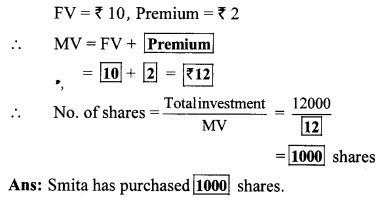## Maharashtra State Board Class 10 Maths Solutions Chapter 5 Co-ordinate Geometry Practice Set 5.3

Practice Set 5.3 Geometry Class 10 Question 1. Angles made by the line with the positive direction of X-axis are given. Find the slope of these lines.
i. 45°
ii. 60°
iii. 90°
Solution:
i. Angle made with the positive direction of
X-axis (θ) = 45°
Slope of the line (m) = tan θ
∴ m = tan 45° = 1
∴ The slope of the line is 1.

ii. Angle made with the positive direction of X-axis (θ) = 60°
Slope of the line (m) = tan θ
∴ m = tan 60° = $$\sqrt { 3 }$$
∴ The slope of the line is $$\sqrt { 3 }$$.

iii. Angle made with the positive direction of
X-axis (θ) = 90°
Slope of the line (m) = tan θ
∴ m = tan 90°
But, the value of tan 90° is not defined.
∴ The slope of the line cannot be determined.

Practice Set 5.3 Geometry Question 2. Find the slopes of the lines passing through the given points.
i. A (2, 3), B (4, 7)
ii. P(-3, 1), Q (5, -2)
iii. C (5, -2), D (7, 3)
iv. L (-2, -3), M (-6, -8)
v. E (-4, -2), F (6, 3)
vi. T (0, -3), s (0,4)
Solution:
i. A (x1, y1) = A (2, 3) and B (x2, y2) = B (4, 7)
Here, x1 = 2, x2 = 4, y1 = 3, y2 = 7∴ The slope of line AB is 2.

ii. P (x1, y1) = P (-3, 1) and Q (x2, y2) = Q (5, -2)
Here, x1 = -3, x2 = 5, y1 = 1, y2 = -2∴ The slope of line PQ is $$\frac { -3 }{ 8 }$$

iii. C (x1, y1) = C (5, -2) and D (x2, y2) = D (7, 3)
Here, x1 = 5, x2 = 7, y1 = -2, y2 = 3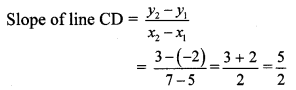∴ The slope of line CD is $$\frac { 5 }{ 2 }$$

iv. L (x1, y1) = L (-2, -3) and M (x2,y2) = M (-6, -8)
Here, x1 = -2, x2 = – 6, y1 = – 3, y2 = – 8∴ The slope of line LM is $$\frac { 5 }{ 4 }$$

v. E (x1, y1) = E (-4, -2) and F (x2, y2) = F (6, 3)
Here,x1 = -4, x2 = 6, y1 = -2, y2 = 3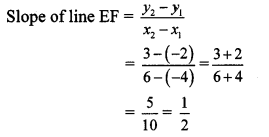∴ The slope of line EF is $$\frac { 1 }{ 2 }$$.

vi. T (x1, y1) = T (0, -3) and S (x2, y2) = S (0, 4)
Here, x1 = 0, x2 = 0, y1 = -3, y2 = 4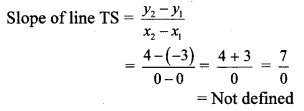∴ The slope of line TS cannot be determined.

5.3.5 Practice Question 3. Determine whether the following points are collinear.
i. A (-1, -1), B (0, 1), C (1, 3)
ii. D (- 2, -3), E (1, 0), F (2, 1)
iii. L (2, 5), M (3, 3), N (5, 1)
iv. P (2, -5), Q (1, -3), R (-2, 3)
v. R (1, -4), S (-2, 2), T (-3,4)
vi. A(-4,4),K[-2,$$\frac { 5 }{ 2 }$$], N (4,-2)
Solution: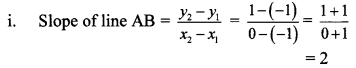∴ slope of line AB = slope of line BC
∴ line AB || line BC
Also, point B is common to both the lines.
∴ Both lines are the same.
∴ Points A, B and C are collinear.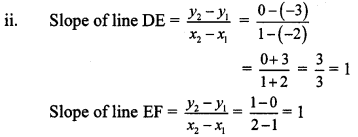∴ slope of line DE = slope of line EF
∴ line DE || line EF
Also, point E is common to both the lines.
∴ Both lines are the same.
∴ Points D, E and F are collinear.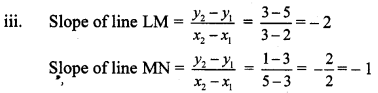∴ slope of line LM ≠ slope of line MN
∴ Points L, M and N are not collinear.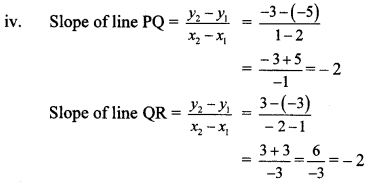∴ slope of line PQ = slope of line QR
∴ line PQ || line QR
Also, point Q is common to both the lines.
∴ Both lines are the same.
∴ Points P, Q and R are collinear.∴ slope of line RS = slope of line ST
∴ line RS || line ST
Also, point S is common to both the lines.
∴ Both lines are the same.
∴ Points R, S and T are collinear.∴ slope of line AK = slope of line KN
∴ line AK || line KN
Also, point K is common to both the lines.
∴ Both lines are the same.
∴ Points A, K and N are collinear.

Practice Set 5.3 Geometry 9th Standard Question 4. If A (1, -1), B (0,4), C (-5,3) are vertices of a triangle, then find the slope of each side.
Solution: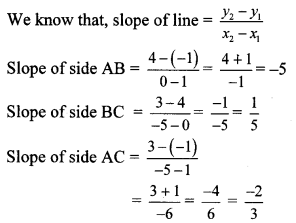∴ The slopes of the sides AB, BC and AC are -5, $$\frac { 1 }{ 5 }$$ and $$\frac { -2 }{ 3 }$$ respectively.

Geometry 5.3 Question 5. Show that A (-4, -7), B (-1, 2), C (8, 5) and D (5, -4) are the vertices of a parallelogram.
Proof: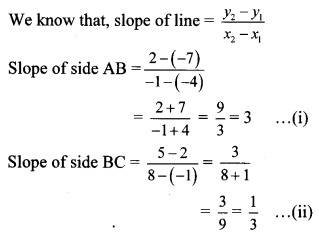∴ Slope of side AB = Slope of side CD … [From (i) and (iii)]
∴ side AB || side CD
Slope of side BC = Slope of side AD … [From (ii) and (iv)]
∴ side BC || side AD
Both the pairs of opposite sides of ꠸ABCD are parallel.
꠸ABCD is a parallelogram.
Points A(-4, -7), B(-1, 2), C(8, 5) and D(5, -4) are the vertices of a parallelogram.

Question 6.
Find k, if R (1, -1), S (-2, k) and slope of line RS is -2.
Solution:
R(x1, y1) = R (1, -1), S (x2, y2) = S (-2, k)
Here, x1 = 1, x2 = -2, y1 = -1, y2 = kBut, slope of line RS is -2. … [Given]
∴ -2 = $$\frac { k+1 }{ -3 }$$
∴ k + 1 = 6
∴ k = 6 – 1
∴ k = 5

5.3 Class 10 Question 7. Find k, if B (k, -5), C (1, 2) and slope of the line is 7.
Solution:
B(x1, y1) = B (k, -5), C (x2, y2) = C (1, 2)
Here, x1 = k, x2 = 1, y1 = -5, y2 = 2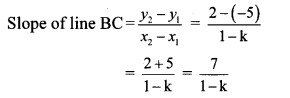But, slope of line BC is 7. …[Given]
∴ 7 = $$\frac { 7 }{ 1-k }$$
∴ 7(1 – k) = 7
∴ 1 – k = $$\frac { 7 }{ 7 }$$
∴ 1 – k = 1
∴ k = 0

Question 8.
Find k, if PQ || RS and P (2, 4), Q (3, 6), R (3,1), S (5, k).
Solution: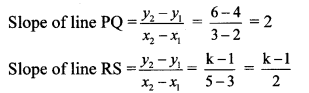But, line PQ || line RS … [Given]
∴ Slope of line PQ = Slope of line RS
∴ 2 = $$\frac { k-1 }{ 2 }$$
∴ 4 = k – 1
∴ k = 4 + 1
∴ k = 5

## Maharashtra State Board Class 10 Maths Solutions Chapter 5 Co-ordinate Geometry Practice Set 5.1

Practice Set 5.1 Geometry Class 10 Question 1. Find the distance between each of the following pairs of points.
i. A (2, 3), B (4,1)
ii. P (-5, 7), Q (-1, 3)
iii. R (0, -3), S (0,$$\frac { 5 }{ 2 }$$)
iv. L (5, -8), M (-7, -3)
v. T (-3, 6), R (9, -10)
vi. W($$\frac { -7 }{ 2 }$$,4), X(11, 4)
Solution:
i. Let A (x1, y1) and B (x2, y2) be the given points.
∴ x1 = 2, y1 = 3, x2 = 4, y2 = 1
By distance formula,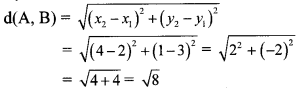∴ d(A, B) = 2$$\sqrt { 2 }$$ units
∴ The distance between the points A and B is 2$$\sqrt { 2 }$$ units.

ii. Let P (x1, y1 ) and Q (x2, y2) be the given points.
∴ x1 = -5, y1 = 7, x2 = -1, y2 = 3
By distance formula,∴ d(P, Q) = 4$$\sqrt { 2 }$$ units
∴ The distance between the points P and Q is 4$$\sqrt { 2 }$$ units.

iii. Let R (x1, y1) and S (x2, y2) be the given points.
∴ x1 = 0, y1 = -3, x2 = 0, y2 = $$\frac { 5 }{ 2 }$$
By distance formula,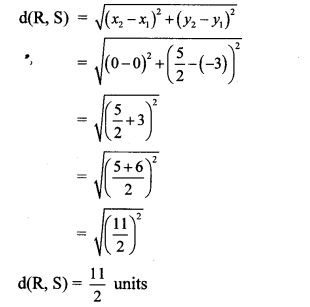∴ d(R, S) = $$\frac { 11 }{ 2 }$$ units
∴ The distance between the points R and S is $$\frac { 11 }{ 2 }$$ units.

iv. Let L (x1, y1) and M (x2, y2) be the given points.
∴ x1 = 5, y1 = -8, x2 = -7, y2 = -3
By distance formula,∴ d(L, M) = 13 units
∴ The distance between the points L and M is 13 units.

v. Let T (x1,y1) and R (x2, y2) be the given points.
∴ x1 = -3, y1 = 6,x2 = 9,y2 = -10
By distance formula,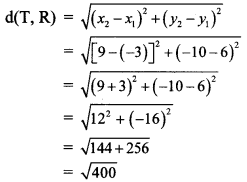∴ d(T, R) = 20 units
∴ The distance between the points T and R 20 units.

vi. Let W (x1, y1) and X (x2, y2) be the given points.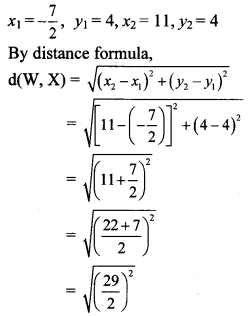∴ d(W, X) = $$\frac { 29 }{ 2 }$$ units
∴ The distance between the points W and X is $$\frac { 29 }{ 2 }$$ units.

Practice Set 5.1 Geometry 10th Question 2. Determine whether the points are collinear.
i. A (1, -3), B (2, -5), C (-4, 7)
ii. L (-2, 3), M (1, -3), N (5, 4)
iii. R (0, 3), D (2, 1), S (3, -1)
iv. P (-2, 3), Q (1, 2), R (4, 1)
Solution:
i. By distance formula,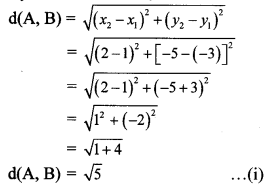∴ d(A, B) = $$\sqrt { 5 }$$ …(i)
d(A, B) + d(A, C)= $$\sqrt { 5 }$$ + 5$$\sqrt { 5 }$$ = 6$$\sqrt { 5 }$$
∴ d(A, B) + d(A, C) = d(B, C) … [From (ii)]
∴ Points A, B and C are collinear.

ii. By distance formula,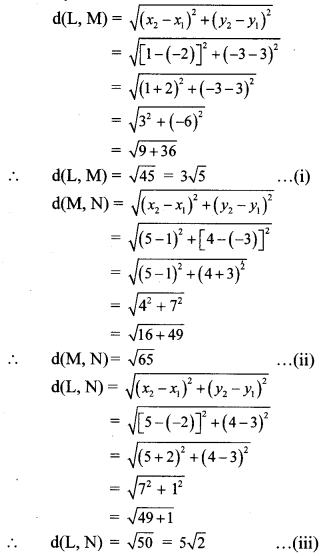d(L, M) + d(L, N) = 3$$\sqrt { 5 }$$ + 5$$\sqrt { 2 }$$ ≠ $$\sqrt { 65 }$$
∴ d(L, M) + d(L, N) ≠ d(M, N) … [From (ii)]
∴ Points L, M and N are not collinear.

iii. By distance formula,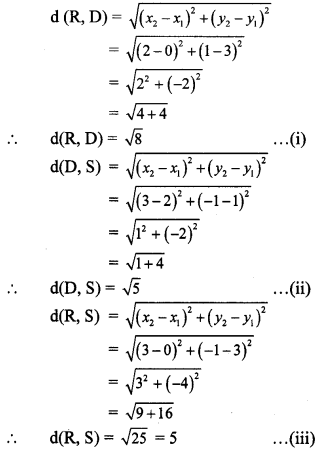∴ d(R, D) + d(D, S) = $$\sqrt { 8 }$$ + $$\sqrt { 5 }$$ ≠ 5
∴ d(R, D) + d(D, S) ≠ d(R, S) … [From (iii)]
∴ Points R, D and S are not collinear.

iv. By distance formula,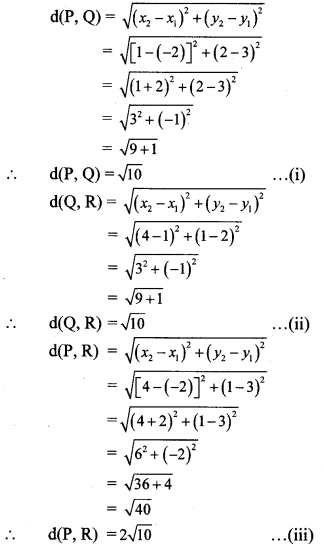d(P, Q) + d(Q, R) = $$\sqrt { 10 }$$ + $$\sqrt { 10 }$$ = 2$$\sqrt { 10 }$$
∴ d(P, Q) + d(Q, R) = d(P, R) … [From (iii)]
∴ Points P, Q and R are collinear.

Coordinate Geometry Class 10 Practice Set 5.1 Question 3. Find the point on the X-axis which is equidistant from A (-3,4) and B (1, -4).
Solution:
Let point C be on the X-axis which is equidistant from points A and B.
Point C lies on X-axis.
∴ its y co-ordinate is 0.
Let C = (x, 0)
C is equidistant from points A and B.
∴ AC = BC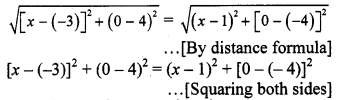∴ (x + 3)2 + (-4)2 = (x- 1)2 + 42
∴ x2 + 6x + 9 + 16 = x2 – 2x + 1 + 16
∴ 8x = – 8
∴ x = – $$\frac { 8 }{ 8 }$$ = -1
∴ The point on X-axis which is equidistant from points A and B is (-1,0).

10th Geometry Practice Set 5.1 Question 4. Verify that points P (-2, 2), Q (2, 2) and R (2, 7) are vertices of a right angled triangle.
Solution:
Distance between two points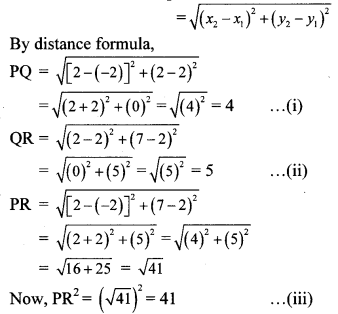Consider, PQ2 + QR2 = 42 + 52 = 16 + 25 = 41 … [From (i) and (ii)]
∴ PR2 = PQ2 + QR2 … [From (iii)]
∴ ∆PQR is a right angled triangle. … [Converse of Pythagoras theorem]
∴ Points P, Q and R are the vertices of a right angled triangle.

Question 5.
Show that points P (2, -2), Q (7, 3), R (11, -1) and S (6, -6) are vertices of a parallelogram.
Proof:
Distance between two points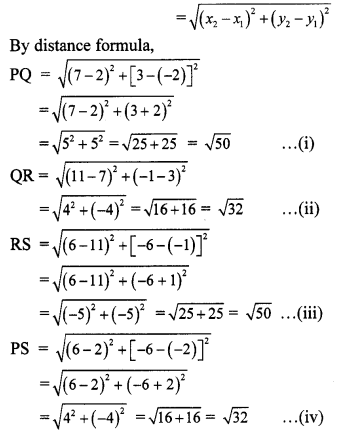PQ = RS … [From (i) and (iii)]
QR = PS … [From (ii) and (iv)]
A quadrilateral is a parallelogram, if both the pairs of its opposite sides are congruent.
∴ □ PQRS is a parallelogram.
∴ Points P, Q, R and S are the vertices of a parallelogram.

Question 6.
Show that points A (-4, -7), B (-1, 2), C (8, 5) and D (5, -4) are vertices of rhombus ABCD.
Proof:
Distance between two points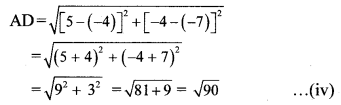∴ AB = BC = CD = AD …[From (i), (ii), (iii) and (iv)]
In a quadrilateral, if all the sides are equal, then it is a rhombus.
∴ □ ABCD is a rhombus.
∴ Points A, B, C and D are the vertices of rhombus ABCD.

Practice Set 5.1 Question 7. Find x if distance between points L (x, 7) and M (1,15) is 10.
Solution:
X1 = x, y1 = 7, x2 = 1, y2 = 15
By distance formula,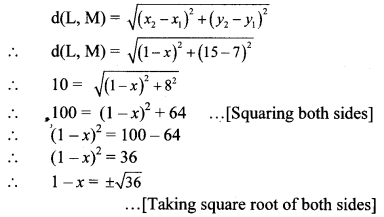∴ 1 – x = ± 6
∴ 1 – x = 6 or l – x = -6
∴ x = – 5 or x = 7
∴ The value of x is – 5 or 7.

Geometry 5.1 Question 8. Show that the points A (1, 2), B (1, 6), C (1 + 2$$\sqrt { 3 }$$, 4) are vertices of an equilateral triangle.
Proof:
Distance between two points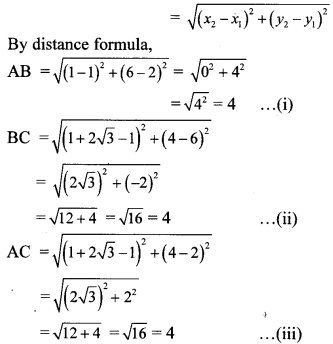∴ AB = BC = AC … [From (i), (ii) and (iii)]
∴ ∆ABC is an equilateral triangle.
∴ Points A, B and C are the vertices of an equilateral triangle.

Maharashtra Board Class 10 Maths Chapter 5 Coordinate Geometry Intext Questions and Activities

Question 1.
In the figure, seg AB || Y-axis and seg CB || X-axis. Co-ordinates of points A and C are given. To find AC, fill in the boxes given below. (Textbook pa. no. 102)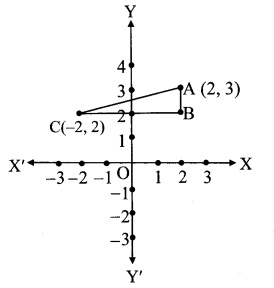Solution:
In ∆ABC, ∠B = 900
∴ (AB)2 + (BC)2 = [(Ac)2 …(i) … [Pythagoras theorem]
seg CB || X-axis
∴ y co-ordinate of B = 2
seg BA || Y-axis
∴ x co-ordinate of B = 2
∴ co-ordinate of B is (2, 2) = (x1,y1)
co-ordinate of A is (2, 3) = (x2, Y2)
Since, AB || to Y-axis,
d(A, B) = Y2 – Y1
d(A,B) = 3 – 2 = 1
co-ordinate of C is (-2,2) = (x1,y1)
co-ordinate of B is (2, 2) = (x2, y2)
Since, BC || to X-axis,
d(B, C) = x2 – x1
d(B,C) = 2 – -2 = 4
∴ AC2 = 12 + 42 …[From (i)]
= 1 + 16 = 17
∴ AC = $$\sqrt { 17 }$$ units …[Taking square root of both sides]

## Maharashtra State Board Class 9 Maths Solutions Chapter 5 Linear Equations in Two Variables Practice Set 5.2

Question 1.
In an envelope there are some ₹5 notes and some ₹10 notes. Total amount of these notes together is ₹350. Number of ₹5 notes are less by 10 than twice the number of ₹10 notes. Then find the number of ₹5 and ₹10 notes.
Solution:
Let the number of ₹5 notes be ‘x’ and the number of ₹10 notes be ‘y’
Total amount of x notes of ₹ 5 = ₹ 5x
Total amount ofy notes of ₹ 10 = ₹ 10y
∴ Total amount = 5x + 10y
According to the first condition,
total amount of the notes together is ₹350.
∴ 5x + 10y = 350 …(i)
According to the second condition,
Number of ₹ 5 notes are less by 10 than twice the number of ₹ 10 notes.
∴ x = 2y – 10
∴ x – 2y = -10 …..(ii)
Multiplying equation (ii) by 5,
5x – 10y = -50 …(iii)
5x + 10y =350
+ 5x – 10y = -50
10x =300
∴ x = $$\frac { 300 }{ 10 }$$
∴ x = 30
Substituting x = 30 in equation (ii),
x – 2y = -10
30 – 2y = -10
∴ 30 + 10 = 2y
∴ 40 = 2y
∴ y = $$\frac { 40 }{ 2 }$$
∴ y = 20
There are 30 notes of ₹ 5 and 20 notes of ₹ 10 in the envelope.

Question 2.
The denominator of a fraction is 1 less than twice its numerator. If 1 is added to numerator and denominator respectively, the ratio of numerator to denominator is 3 : 5. Find the fraction.
Solution:
Let the numerator of the fraction be ‘x’ and its denominator be ‘y’.
Then, the required fraction is $$\frac { x }{ y }$$ .
According to the first condition,
the denominator is 1 less than twice its numerator.
∴ y = 2x – 1
∴ 2x – y = 1 …(i)
According to the second condition,
if 1 is added to the numerator and the denominator, the ratio of numerator to denominator is 3 : 5.
∴ $$\frac { x+1 }{ y+1 }$$ = $$\frac { 3 }{ 5 }$$
∴ y + 1 = 5
∴ 5(x + 1) = 3(y + 1)
∴ 5x + 5 = 3y + 3
∴ 5x – 3y = 3 – 5
∴ 5x – 3y = -2 ……(ii)
Multiplying equation (i) by 3,
6x – 3y = 3 …(iii)
Subtracting equation (ii) from (iii),Substituting x = 5 in equation (i),
∴ 2x – y = 1
∴ 2(5) – y = 1
∴ 10 – y = 1
∴ y= 10 – 1 =9
∴ The required fraction is $$\frac { 5 }{ 9 }$$.

Question 3.
The sum of ages of Priyanka and Deepika is 34 years. Priyanka is elder to Deepika by 6 years. Then find their present ages.
Solution:
Let the present age of Priyanka be ‘x’ years and that of Deepika be ‘y’ years.
According to the first condition,
Priyanka’s age + Deepika’s age = 34 years
∴ x + y = 34 …(i)
According to the second condition,
Priyanka is elder to Deepika by 6 years.
∴ x =y + 6
∴ x – y = 6 …..(ii)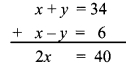∴ x = 20
Substituting x = 20 in equation (i),
x + y = 34
∴ 20 + y = 34
∴ y = 34 -20= 14
∴ The present age of Priyanka is 20 years and that of Deepika is 14 years.

Question 4.
The total number of lions and peacocks in a certain zoo is 50. The total number of their legs is 140. Then find the number of lions and peacocks in the zoo.
Solution:
Let the number of lions in the zoo be ‘x’ and the number of peacocks be ‘y’.
According to the first condition,
the total number of lions and peacocks is 50.
∴ x + y = 50 …(i)
Lion has 4 legs and Peacock has 2 legs.
According to the second condition,
the total number of their legs is 140.
∴ 4x + 2y = 140
Dividing both sides by 2,
2x + y = 70 …(ii)
Subtracting equation (i) from (ii),Substituting x = 20 in equation (i),
x + y = 50
∴ 20 + y = 50
∴ y = 50 – 20 = 30
∴ The number of lions and peacocks in the zoo are 20 and 30 respectively.

Question 5.
Sanjay gets fixed monthly income. Every year there is a certain increment in his salary. After 4 years, his monthly salary was ₹ 4500 and after 10 years his monthly salary became ₹ 5400, then find his original salary and yearly increment.
Solution:
Let the original salary of Sanjay be ₹ ‘x’ and his yearly increment be ₹ ‘y’.
According to the first condition, after 4 years his monthly salary was ₹ 4500
∴ x + 4y = 4500 …..(i)
According to the second condition,
after 10 years his monthly salary became ₹ 5400
∴ x + 10y = 5400 …(ii)
Subtracting equation (i) from (ii),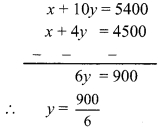∴ y = 150
Substituting y = 150 in equation (i),
x + 4y = 4500
∴ x +4(150) = 4500
∴ x + 600 = 4500
∴ x = 4500 – 600 = 3900
∴ The original salary of Sanjay is ₹ 3900 and his yearly increment is ₹ 150.

Question 6.
The price of 3 chairs and 2 tables is ₹ 4500 and price of 5 chairs and 3 tables is ₹ 7000, then find the price of 2 chairs and 2 tables.
Solution:
Let the price of one chair be ₹ ‘x’ and that of one table be ₹ ‘y’.
According to the first condition,
the price of 3 chairs and 2 tables is ₹ 4500
∴ 3x + 2y = 4500 ,..(i)
According to the second condition, the price of 5 chairs and 3 tables is ? 7000
∴ 5x + 3y = 7000 …(ii)
Multiplying equation (i) by 3,
9x + 6y = 13500 ….(iii)
Multiplying equation (ii) by 2,
10x + 6y= 14000 …(iv)
Subtracting equation (iii) from (iv),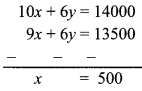Substituting x = 500 in equation (i),
3x + 2y = 4500
∴ 3(500)+ 2y = 4500
∴ 1500 + 2y = 4500
∴ 2y = 4500- 1500
∴ 2y = 3000
∴ y = $$\frac { 3000 }{ 2 }$$
∴ y = 1500
∴ Price of 2 chairs and 2 tables = 2x + 2y
= 2(500)+ 2(1500)
= 1000 + 3000 = ₹ 4000
∴ The price of 2 chairs and 2 tables is ₹ 4000.

Question 7.
The sum of the digits in a two-digit number is 9. The number obtained by interchanging the digits exceeds the original number by 27. Find the two-digit number.
Solution:
Let the digit in unit’s place be ‘x’ and the digit in ten’s place be ‘y’.According to the first condition.
the sum of the digits in a two-digit number is 9
x + y = 9 …(i)
According to the second condition,
the number obtained by interchanging the digits exceeds the original number by 27
∴ 10x + y = 10y + x + 27
∴ 10x – x + y – 10y = 27
∴ 9x – 9y = 27
Dividing both sides by 9,
x – y = 3 …….(ii)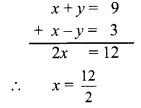∴ x = 6
Substituting x = 6 in equation (i),
x + y = 9
∴ 6 + y = 9
∴ y = 9 – 6 = 3
∴ Original number = 10y + x = 10(3)+ 6
= 30 + 6 = 36
∴ The two digit number is 36.

Question 8.
In ∆ABC, the measure of ∠A is equal to the sum of the measures of ∠B and ∠C. Also the ratio of measures of ∠B and ∠C is 4 : 5. Then find the measures of angles of the triangle.
Solution:
Let the measure of ∠B be ‘x°’ and that of ∠C be ‘y°’.
According to the first condition,
m∠A = m∠B + m∠C
∴ m∠A = x° + y°
In AABC,
m∠A + m∠B + m∠C = 180° …[Sum of the measures of the angles of a triangle is 180°]
∴ x + y + x + y = 180 ,
∴ 2x + 2y = 180
Dividing both sides by 2,
x + y = 90 …(i)
According to the second condition,
the ratio of the measures of ∠B and ∠C is 4 : 5.
∴ $$\frac { x }{ y }$$ = $$\frac { 4 }{ 5 }$$
∴ 5x = 4y
∴ 5x – 4y = 0 …….(ii)
Multiplying equation (i) by 4,
4x + 4y = 360 …(iii)∴ x = 40
Substituting x = 40 in equation (i),
x + y = 90
∴ 40 + y = 90
∴ y = 90 – 40
∴ y = 50
∴ m∠A = x° + y° = 40° + 50° = 90°
∴ The measures of ∠A, ∠B and ∠C are 90°, 40°, and 50° respectively.

Question 9.
Divide a rope of length 560 cm into 2 parts such that twice the length of the smaller part is equal to $$\frac { 1 }{ 3 }$$ of the larger part. Then find the length of the larger part.
Solution:
Let the length of the smaller part of the rope be ‘x’ cm and that of the larger part be ‘y’ cm.
According to the first condition,
total length of the rope is 560 cm.
∴ x + y = 560 …(i)
Twice the length of the smaller part = 2x
$$\frac { 1 }{ 3 }$$rd length of the larger part = $$\frac { 1 }{ 3 }$$y
According to the second condition,
2x = $$\frac { 1 }{ 3 }$$ 3
∴ 6x = y
∴ 6x – y = 0 ……(ii)∴ x = 80
Substituting x = 80 in equation (ii),
6x – y = 0
∴ 6(80) – y = 0
∴ 480 – y = 0
∴ y = 480
∴ The length of the larger part of the rope is 480 cm.

Question 10.
In a competitive examination, there were 60 questions. The correct answer would carry 2 marks, and for incorrect answer 1 mark would be subtracted. Yashwant had attempted all the questions and he got total 90 marks. Then how many questions he got wrong?
Solution:
Let us suppose that Yashwant got ‘x’ questions right and ‘y’ questions wrong.
According to the first condition, total number of questions in the examination are 60.
∴ x + y = 60 …(i)
Yashwant got 2 marks for each correct answer and 1 mark was deducted for each wrong answer.
∴ He got 2x – y marks.
According to the second condition,
he got 90 marks.
2x – y = 90 … (ii)∴ x = 50
Substituting x = 50 in equation (i),
50 + y = 60
∴ y = 60 – 50 = 10
∴ Yashwant got 10 questions wrong.

Maharashtra Board Class 9 Maths Chapter 5 Linear Equations in Two Variables Practice Set 5.2 Intext Questions and Activities

Question 1.
The population of a certain town was 50,000. In a year, male population was increased by 5% and female population was increased by 3%. Now the population became 52020. Then what was the number of males and females in the previous year? (Textbook pg. no. 89)
Solution:
Step 1: Read the given word problem carefully and try to understand it.

Step 2: Make assumptions using two variables x and y.
Let the number of males in previous year be
‘x’ and the number of females be ‘y’.

Step 3: From the given information, form mathematical statements using the above variables.
According to the first condition,
the total population of town was 50,000.
∴ x + y = 50000 …(i)
Male population increased by 5%.
∴ Number of males = x + 5% of x , 5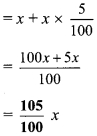Female population increased by 3%.
∴ Number of females = y + 3% of yAccording to the second condition,
in a year population became 52020
∴ $$\frac{105}{100} x+\frac{103}{100} y=52020$$
∴ 105 x + 103 y = 5202000 …(ii)
Multiplying equation (i) by 103,
103 x + 103 y = 5150000 …(iii)

Step 4: Here, we use elimination method.
Subtracting equation (iii) from (ii),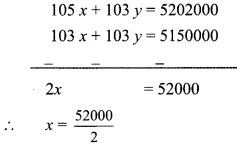∴ x = 26000
Substituting x = 26000 in equation (i),
∴ 26000 + y = 50000
∴ y = 50000 – 26000
∴ y = 24000
∴ Number of males = x = 26000
∴ Number of females = y = 24000